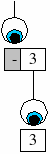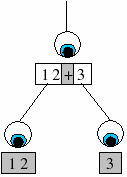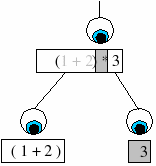# Guide to the Agent-Oriented Parser

## Getting Started

### Parser concepts

In order to learn to use the Agent-oriented Parser in Eden we must put aside our prior knowledge of parsing. Conventional parsers read each input character, one at a time, and not until the entire string is read can any meaning be derived. Instead, it is useful to think about how we, as humans, read languages (natural or otherwise). When we read sentences, we do not remember each character, or even each word, but our brains register the important words. From this we are able to derive meaning.

An agent is an independent entity capable of acting on and interacting with an environment. In the Agent-oriented Parser, the agents have a set of rules (a grammar) with which to parse any input. Each agent will take an input string and a rule, with which it will determine whether the rule can be applied. If the rule is applied, then more agents could be generated to work on substrings. The agent will fail if it cannot apply the rule.

A rule specifies a string that must be observed in the input string. For example, an agent might have the input ‘1+2’ and the most important string it must find could be ‘+’. When the agent observes a match, it will pass the remaining substrings (if any) to new agents. These are called child agents.The diagram shows how agents could parse a string. In this example the input is an arithmetic expression and the rules are such that the expression is parsed using standard operator precedence.

### Writing notations

A notation is defined as a set of rules, which specify the behaviour of our agents. A rule in %aop has the following syntax:

`<RULE_NAME> = PATTERN_DESCRIPTION            (The pattern description, Required.)            : do {EDEN_ACTIONS} NOW_OR_LATER (Eden actions, Optional.)            : ignore [IGNORE_LIST]           (Any ignore blocks, Optional.)            | <NEXT_RULE_NAME>;              (The next rule to try, Optional.)`

%aop uses the same namespace as Eden (so RULE_NAME and NEXT_RULE_NAME are Eden observables). A pattern description contains the pattern that the agent is trying to find (or observe) in the input. It also constains information about which rules to use on any substrings. This will be explained in detail later. Every rule must have a pattern description, this is the minimum that a rule can contain. It is likely that most rule definitions will have a 'next rule' (also called the 'fail') clause, such that if the agent fails to match its pattern, then it will try another rule.

### Developing a calculator notation

A simple calculator is able to accept digits and arithmetic operators. It will calculate the result of the input expression. Calculators also allow nested expressions using brackets.

Recall that our Agent-oriented Parser uses an agent to observe a string in the input. The simplest agents therefore match the input entirely. On a calculator, the user can input just a digit, and the result will be that same digit.

 The first operation we will be introduced to is "literal". This operation attempts to match the entire input string (see diagram right). It does not create any child agents. Lets define a rule that matches the digit ‘1’:`<number1> = "1";`

To invoke the parser, you must supply an input string and a starting rule. The only input our rule should match is ‘1’, so to test this run the parser:

`aop_parse("1", "number1", []);`

It should accept. The first parameter is the input and the second is the starting rule. Try other inputs to get the parser to reject.

Our language is not very powerful, being only able to accept the digit ‘1’. Now we will introduce the fail clause. We can get our parser to try another rule should the operation fail:

```<number1> = "1" | <number2>;
<number2> = "2";
```

The language will now accept either of the digits ‘1’ or ‘2’. We could extend this method to accept all the digits.

### Iteratively developing the model

Now that our calculator can parse digits, we will introduce operators to the input. This is an important concept in Empirical Modelling, the ability to build up a model in an iterative fashion.

Our current model allows us to parse positive numbers, so an obvious extension is to allow negative numbers too. We can define an integer as a number with an optional minus symbol (-) in front of it.

 The next agent operation we shall introduce is "prefix". This operation matches a string at the beginning of the input. If a match is made then a child agent is created with its input as the unmatched part of the agents input (see diagram right). This operation can be used to detect a minus symbol at the beginning of our number:```<term> = "-" <number1> | <number1>;
```

Notice that we use the same rule for the child agent and the fail clause. The parser will try to match a minus sign at the start of the input, if it matches then it will match the remainder of the input as a number, else it will match the entire input as a number.

A similar operation is "suffix" which does exactly the same as "prefix", but at the end of a string. An example of using suffix is to remove the semi-colon from the end of a string (i.e. to parse a statement in C/Java/Eden)

The parser should now accept any positive and negative numbers. We shall now look at how we can parse arithmetic operations (e.g. +, -, *, /). As always we will begin with something simple and build the model up. We will first try to parse expressions containing only additions of terms, where our terms are the integers we learnt to parse above.

 This is where the most powerful agent operation comes in. The "pivot" operation searches the input (left to right) for a specified string. If it finds a match, then it creates two child agents, one for the left substring and one for the right substring (as shown in the diagram on the right). Our parser would pivot on the addition sign:`<expr> = <expr> "+" <expr> | <term>;`

An "expr" agent is looking for an addition sign, and if it finds one it creates two children that also search for expressions. If the agent finds no addition sign on the input, then the fail clause specifies that the input must be a term.

In order for our parser to recognise expression containing other operators it is necessary for us to have a rule for each string to be matched:

```<expr>  = <expr> "+" <expr> | <expr2><expr2> = <expr> "-" <expr> | <expr3><expr3> = <expr> "*" <expr> | <expr4><expr4> = <expr> "/" <expr> | <term>;
```

Notice that we search for operators in their reverse precedence order. This can be explain by taking an example, say the input is ‘1+2*3’. First we would pivot on the addition sign giving us two substrings ‘1’ and ‘2*3’. We have broken the calculation down into two sub-calculations which we will add together later. The deepest level will get calculated first, which in this example is ‘2*3’. Therefore we are observing the rules of precedence correctly.

### Regular expressions

Using just the pivot and literal operations gives you all the power you need to develop languages. However, the rules would be quite cumbersome without regular expressions. The Agent-oriented Parser has 3 other operations that deal with basic regular expressions.

The first is "read_all", which will match any text:

`<match_anything> = *;`

### Perl-style regular expressions

The above regular expressions lack power, for example, you cannot specify rules for real numbers or identifiers. Our definition of a "number" will accept the empty string – not desirable in most cases!

Three more regular expression operations exist in the latest version of the Agent-oriented Parser. These are "literal_re", "prefix_re" and "suffix_re". The first matches the whole input, where as the other 2 match the beginning and the end of the input respectively. The pattern to be matched is a perl-style regular expression. For example, the following correctly parses a number:

`<number> = ([0-9]+);`

More information on perl regular expressions can be found in any good perl book or on the web.

### Blocks

Now we shall look at adding brackets to our notation. This will give our calculator the ability to work out expressions like ‘(1+2)*3’. We have seen how to use the "prefix" and "suffix" operations, so we can use these to parse brackets:

```<expr5> = "(" <expr6> | term;
<expr6> = <expr> ")";
```

This gives us a bit of a problem. Think about the input ‘(1+2)*3’. The first agent will use the pivot operation on the ‘+’, leaving two substrings ‘(1’ and ‘2)*3’. Instead, we really want the parser to pivot on the ‘*’ and break the input into the two smaller expressions ‘(1+2)’ and ‘3’. The parser needs to be sensitive with our brackets.

This is where blocks are a very useful feature of the Agent-oriented Parser. We can define a block and instruct agents to ignore that block.
Here we define a block for parentheses. This block can contain more parentheses because we've allowed the block the same block.

`block bras = "(" to ")" contains [bras];`
 Now for a particular rule we can specify it to ignore blocks. For our calculator notation, we want to ignore any strings between brackets when the agent is looking for an arithmetic operator (+,-,*,/). We add an ignore clause to our rule:`<expr7> = <expr> "+" <expr> : ignore [bras] | <expr2>;`

This behaviour is demonstrated in the diagram (right). The string within the brackets is ‘greyed-out’ because it is ignored. Hence the most important string to be observed is the multiplication sign (*) and a pivot is made.

It is important to remember that when an agent ‘ignores’ a block it is not removing that block from the input. It is perhaps better described as preserving the block. The agent simply preserves the contents of the block and leaves it for another agent to parse.

### Scripting

A parser that either accepts or rejects an input is of little use unless it produces some output. Our simple calculator needs some way of outputting the result of an expression. This is achieved with agent actions. If an agent does not fail to match the input then it can optionally perform some actions. Each action can be performed before or after the actions of the agent’s children.

The format for including agent actions in a rule definition is similar to the other optional components of a rule. Here we modify our "term" rule by adding an action that prints out some random comment:

`<term> = ([0-9]+) : do {writeln("somerandomcomment");} now;`

The do {...} now construction above is the action declaration. It begins with the "do" tag and the text inside the curly braces  are the Eden commands to execute. The command is followed by  either "now" or "later" depending on whether the command will be executed before or after the child agents.

An agent has some data associated with it that can used in its actions. Each agent also has a unique variable associated with it. This agent data can be substituted into the command string using the following:

\$i = the input string to the agent
\$j = the name of a variable containing the input string
\$t = the token/string that was matched by the agent
\$v = the variable name that belongs to the agent
\$s1 = the first substring of the input
\$s2 = the second substring of the input (and so on for 3rd, 4th, ..)
\$p1 = the variable name of the first child agent (parameter 1)
\$p2 = the variable name of the second child agent

Now we can make our "term" rule more useful by adding an action that stores the term value in the agent variable:

`<term> = ([0-9]+) : do {\$v = \$t;} now;`

We would then add actions to our other rules. The "expr" rule can do the addition of the two sub-expressions:

<expr> = <expr> "+" <expr> : do {\$v = \$p1 + \$p2;} later | <term>;

Take a look at the final calculator notation at the end of the document for more examples of agent actions.

Note: The dollar sign is a special character in the command string. If you want to print a single dollar sign (\$) in your command string then you must follow it by another dollar sign ("\$\$" will produce a single dollar in the command string).

### Installing notations

Now that we are happy with our calculator notation, it is probably a good idea to make it more accessible. We can install new notations into the Eden environment, which can then be used in scripts as you would existing notations (e.g. %eden, %donald, %scout). In tkeden this will add a radio button for our new notation to the environment.

To do this we define the notation using the notation keyword:

`notation %mynotation = <calc> split on "\n";`

This installs a new notation called %mynotation into EDEN. Input will be split on newline characters and will use the <calc> rule to begin the parsing.

Notations must begin with a percent (%) character.

You can now switch to the new notation by typing %mynotation. Do not forget to switch back to %eden for Eden code!

This document was originally prepared by Anthony Harfield as part of his third year project (See the original), and updated to relate to the new AOP Notation by Charles Care (November 2005).